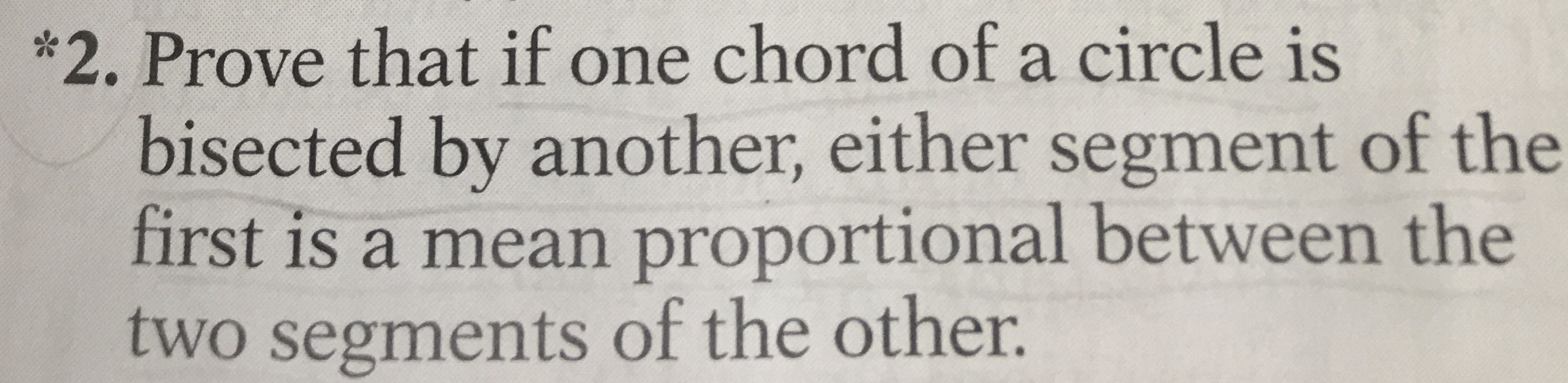# *2. Prove that if one chord of a circle is bisected by another, either segment of the first is a mean proportional between the two segments of the other.

Question

Please answer in a two-column proof-statements and reasons. **Also, it should have ONLY one given (statement).

It is about lines proportional. The question is in the picture. *The question is completely clear, so please don't reject the question.*

*Maybe, if you could, please use some of these reasons to prove the statements true. (Those below are the reasons  for the statements if you want to use)*

Definition of ~ triangles

Definition of perimeter

Definition of median

Definition of midpoint

Multiplication

Division

If 4 quantities are in proportion, then like powers are in proportion.

Subtraction Transformation

Alternation Transformation

Pythagorean Theorem

If 2 angles have their sides perpendicular, right side to right side and left side to left side, the angles are equal.

In a series of equal ratios, the sum of the antecedents is to the sum of the consequents as any antecedent is to its consequent.

Angles inscribed in the same segment or equal segments are equal.

If a line is drawn parallel to the base of a triangle, it cuts off a triangle similar to the given triangle.

Two isosceles triangles are similar if any angle of one equals the corresponding angle of the other.

C.A.S.T.E. - corresponding angles of similar triangles are equal

C.S.S.T.P. - corresponding sides of similar triangles are proportional

Theorem 57- If two triangles have the three angles of one equal respectively to the three angles of the other, then the triangles are similar

Corollary 57-1 If two angles of one triangle are equal respectively to two angles of another, then the triangles are similar. (a.a.)

Corollary 57-2 Two right triangles are similar if an acute angle of one is equal to an acute angle of the other.

Theorem 58-If two triangles have two pairs of sides proportional and the included angles equal respectively, then the two triangles are similar. (s.a.s.)

Corollary 58-1 If the legs of one right triangle are proportional to the legs of another, the triangles are similar. (l.l.)

Theorem 59- If two triangles have their sides respectively proportional, then the triangles are similar. (s.s.s.)

Theorem 60- If two parallels are cut by three or more transversals passing through a common point, then the corresponding segments of the parallels are proportional.

Theorem 61-If in a right triangle the perpendicular is drawn from the vertex of the right angle to the hypotenuse

Corollary 61-1 If a perpendicular is dropped from any point on a circle upon a diameter, then the perpendicular is the mean proportional between the segments of the diameter.

Theorem 62-The square of the hypotenuse of a right triangle is equal to the sum of the squares of the legs.

Corollary 62-1 The difference of the square of the hypotenuse and the square of one leg equals the square of the other leg.

Theorem 63- If two chords intersect within a circle, then the product of the segments of one is equal to the product of the segments of the other.

Corollary 63-1 The product of the segments of any chord through a fixed point within a circle is constant.

Theorem 64-If from a point outside a circle, a tangent and a secant are drawn to the circle, then the tangent is the mean proportional between the secant and its external segment.

Corollary 64-1 The product of any secant from a fixed point outside the circle and its external segment is constant.

Corollary 64-2 If two or more secants are drawn to a circle from a fixed point outside the circle, then the product of one secant and its external segment is equal to the product of any other secant and its external segment.help_outlineImage Transcriptionclose*2. Prove that if one chord of a circle is bisected by another, either segment of the first is a mean proportional between the two segments of the other. fullscreen

### Want to see this answer and more?

Experts are waiting 24/7 to provide step-by-step solutions in as fast as 30 minutes!*

*Response times vary by subject and question complexity. Median response time is 34 minutes and may be longer for new subjects.
Tagged in
MathGeometry

### Circles